Courses

# CAM And Balancing Of Rigid Rotors And Field Balancing - MCQ Test 1

## 15 Questions MCQ Test Mock Test Series - Mechanical Engineering (ME) for GATE 2020 | CAM And Balancing Of Rigid Rotors And Field Balancing - MCQ Test 1

Description
This mock test of CAM And Balancing Of Rigid Rotors And Field Balancing - MCQ Test 1 for Mechanical Engineering helps you for every Mechanical Engineering entrance exam. This contains 15 Multiple Choice Questions for Mechanical Engineering CAM And Balancing Of Rigid Rotors And Field Balancing - MCQ Test 1 (mcq) to study with solutions a complete question bank. The solved questions answers in this CAM And Balancing Of Rigid Rotors And Field Balancing - MCQ Test 1 quiz give you a good mix of easy questions and tough questions. Mechanical Engineering students definitely take this CAM And Balancing Of Rigid Rotors And Field Balancing - MCQ Test 1 exercise for a better result in the exam. You can find other CAM And Balancing Of Rigid Rotors And Field Balancing - MCQ Test 1 extra questions, long questions & short questions for Mechanical Engineering on EduRev as well by searching above.
QUESTION: 1

### In a plate cam mechanism with reciprocating roller follower, the follower has a constant acceleration in the case of   [GATE-1993]

Solution:

For uniform acceleration and retardation, the velocity of the follower must change at a constant rate and hence the velocity diagram of the follower consists of sloping straight lines. The velocity diagram represents everywhere the slope of the displacement diagram, the later must be a curve whose slope changes at a constant rate. Hence the displacement diagram consists of double parabola.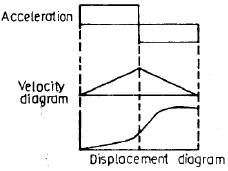QUESTION: 2

### A rotating disc of 1 m diameter has two eccentric masses of 0.5 kg each at radii of 50 mm and 60 mm at angular positions of 0°and 150°, respectively. a balancing mass of 0.1 kg is to be used to balance the rotor. What is the radial position of the balancing mass?           [GATE-2005]

Solution: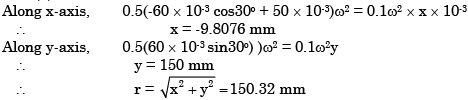QUESTION: 3

### In a cam-follower mechanism, the follower needs to rise through 20 mm during 60o of cam rotation, the first 30o with a constant acceleration and then with a deceleration of the same magnitude. The initial and final speeds of the follower are zero. The cam rotates at a uniform speed of 300 rpm. The maximum speed of the follower is [GATE-2005]

Solution: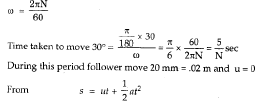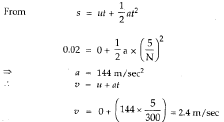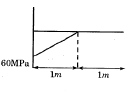QUESTION: 4

What is the condition for dynamic balancing of a shaft-rotor system?

[IES 2007]

Solution:
QUESTION: 5

For a spring-loaded roller-follower driven with a disc cam,

Solution:
QUESTION: 6

A system in dynamic balance implies that

Solution:

A system in dynamic balance implies that the system is also statically balanced.

QUESTION: 7

In a cam design, the rise motion is given by a simple harmonic motion (SHM)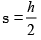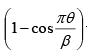where h is total rise, θ is camshaft angle, β is the total angle of the rise interval. The jerk is given by

Solution: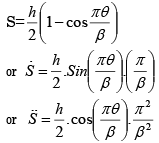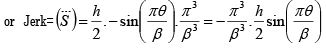QUESTION: 8

A rigid body shown in the Fig. (a) has a mass of 10 kg. It rotates with a uniform angular velocity 'ω'. A balancing mass of 20 kg is attached as shown in Fig. (b). The percentage increase in mass moment of inertia as a result of this addition is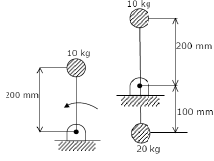Solution: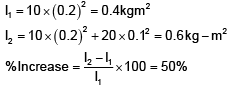QUESTION: 9

The profile of a cam in a particular zone is given by x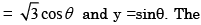normal to the cam profile at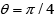is at an angle (with respect to x axis)

Solution: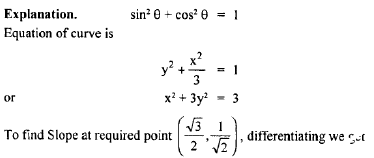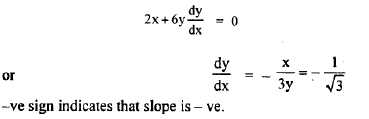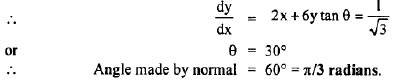QUESTION: 10

Assertion (A): A dynamically balanced system of multiple rotors on a shaft can rotate smoothly at the critical speeds of the system.

Reason (R): Dynamic balancing eliminates all the unbalanced forces and couples from the system.

Solution:
QUESTION: 11

In a circular arc cam with roller follower, the acceleration in any position of the lift would depend only upon

Solution:
QUESTION: 12

Consider the following statements for completely balancing a single rotating mass:

1. Another rotating mass placed diametrically opposite in the same plane balances the unbalanced mass.
2. Another rotating mass placed diametrically opposite in a parallel plane balances the unbalanced mass.
3. Two masses placed in two different parallel planes balance the unbalanced mass.
Which of the above statements is/are correct?

Solution:
QUESTION: 13

Consider the following statements:

Cam followers are generally classified according to
1. the nature of its motion
2. the nature of its surface in contact with the cam
3. the speed of the cam Which of the statements given above are correct?

Solution:
QUESTION: 14

A system of masses rotating in different parallel planes is in dynamic balance if the resultant.

Solution:
QUESTION: 15

In a single spindle automatic lathe two tools are mounted on the turret, one form tool on the front slide and the other, a parting tool on the rear slide. The parting tool operation is much longer than form tool operation and they operate simultaneously (overlap). The number of cams required for this job is

Solution: